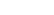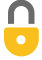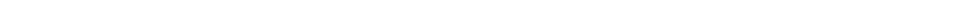Problem 38
Q

# Three students derive the following equations in which $x$ refers to distance traveled, $v$ the speed, $a$ the acceleration $(m/s^2)$, $t$ the time, and the subscript zero $(_0)$ means a quantity at time $t = 0$. Here are their equations:

1. $x = vt^2 + 2at$,
2. $x = v_0 t + \dfrac{1}{2}at^2$, and
3. $x = v_0t + 2at^2$.
Which of these could possibly be correct according to a dimensional check, and why?A
a) no, b) maybe yes, c) maybe yesIn order to watch this solution you need to have a subscription.VIDEO TRANSCRIPT

This is Giancoli Answers with Mr. Dychko. This first formula the distance x equals speed times time squared plus 2 times acceleration times time works out to these following units; we have meters in the left and so that means in the end, we have to have meters in the right if this formula's gonna be correct. So the speed is in meters per second and time squared one of those s squared— seconds squared—and then so one of the seconds here cancels leaving us with meters times seconds which already we know that things aren't gonna work out because we need to have meters but we can look at the second term as well meters per second squared times seconds gives us meters per second which is a speed and either way, this definitely does not work out. Part (b), we have distance on the left and then on the right-hand side we have so that's meters on the left then right-hand side we have speed is meters per second multiplied by seconds and these seconds cancel leaving us with meters so the first term checks; it's good, it's in meters as it should be. Now the second term is in meters per second squared is the units for or dimensions for acceleration multiplied by times squared and these seconds squared's cancel leaving us with meters for the second term and meters plus meters gives meters and so things work out. We have meters on the left, meters on the right; equation in part (b) could be correct. In part (c), we have meters on the left and then on the right we have meters per second times seconds which gives us meters and then we have meters per second squared is the acceleration units times seconds squared for the time squared seconds squared's cancel and seconds here cancel giving us meters plus meters which is meters on the right, meters on the left; this formula could also be correct.

Giancoli Answers, including solutions and videos, is copyright © 2009-2023 Shaun Dychko, Vancouver, BC, Canada. Giancoli Answers is not affiliated with the textbook publisher. Book covers, titles, and author names appear for reference purposes only and are the property of their respective owners. Giancoli Answers is your best source for the 7th and 6th edition Giancoli physics solutions.